DOI: 10.20937/ATM.53131

Received: October 20, 2021; Accepted: June 03, 2022

# Comparative analysis of estimated solar radiation with different learning methods and empirical models

Mehmet Murat Comert1*, Kemal Adem2 and Muberra Erdogan1

1Tokat Gaziosmanpasa University, Faculty of Agriculture, Department of Biosystem Engineering, 60240, Tokat-Turkey

2Sivas University of Science and Technology, Faculty of Engineering and Natural Sciences, Department of Computer Engineering, 58100, Sivas-Turkey

* Corresponding author: mehmetmurat.comert@gop.edu.tr

RESUMEN

La radiación solar, que se utiliza en la modelación hidrológica y agrícola, sistemas de energía solar y en estudios climatológicos, es el elemento más importante de la energía que llega a la tierra. El presente estudio comparó el desempeño de dos ecuaciones empíricas -las ecuaciones de Angstrom y Hargreaves-Samani- y tres modelos de aprendizaje automático -Redes Neuronales Artificiales (ANN), Máquina de Vectores de Soporte (SVM) y Memoria a Largo Corto Plazo (LSTM)-. Se desarrollaron varios modelos de aprendizaje para las variables utilizadas en cada ecuación empírica. En el presente estudio, se utilizaron datos mensuales de seis estaciones en Turquía, tres estaciones que reciben la mayor radiación solar y tres estaciones que reciben la menor radiación solar. En términos de los valores del error cuadrático (MSE), el error cuadrático medio (RMSE), el error absoluto medio (MAE) y el coeficiente de determinación (R2) de cada modelo; el LSTM fue el modelo de aprendizaje más exitoso, seguido de los modelos de aprendizaje automático ANN y SVM, respectivamente. El valor de MAE fue de 2,65 con la ecuación de Hargreaves-Samani y disminuyó a 0,987 con el modelo LSTM mientras que MAE fue de 1,24 en la ecuación de Angstrom y disminuyó a 0,747 con el modelo LSTM. El estudio reveló que el modelo de aprendizaje profundo es más apropiado para usar en comparación con las ecuaciones empíricas, incluso en los casos en que hay datos limitados.

ABSTRACT

Solar radiation, which is used in hydrological and agricultural modeling, agricultural, solar energy systems, and climatological studies, is the most important element of the energy reaching the earth. The present study compared the performance of two empirical equations -Angstrom and Hargreaves-Samani equations- and three machine learning models -Artificial Neural Networks (ANN), Support Vector Machine (SVM), and Long Short-Term Memory (LSTM)-. Various learning models were developed for the variables used in each empirical equation. In the present study, monthly data of six stations in Turkey, three stations receiving the most solar radiation and three stations receiving the lowest solar radiation, were used. In terms of the mean squared error (MSE), root mean squared error (RMSE), mean absolute error (MAE), and determination coefficient (R2) values of each model, the LSTM was the most successful model, followed by ANN and SVM. The MAE value was 2.65 with the Hargreaves-Samani equation and decreased to 0.987 with the LSTM model, while MAE was 1.24 in the Angstrom equation and decreased to 0.747 with the LSTM model. The study revealed that the deep learning model is more appropriate to use than the empirical equations, even in cases with limited data.

Keywords: Solar radiation, Empirical Equations, Machine learning, Deep learning, LSTM model.

1. Introduction

Water resources’s overexploitation associated with global warming affects majorly the quality and amount of water used (Yurekli, 2021). Agricultural water demand is supplied by planning water resources and their correct use. Therefore, evapotranspiration (ET) needs should be determined for sustainable water management. ET is the most important factor that represents the effect of climate parameters on the hydrologic cycle (Pereira et al., 2015). Direct calculation methods of ET are not practical nor economical. Instead, reference evapotranspiration (ET0) is successfully estimated using climate parameters with the development of empirical equations. The Penman-Monteith method is proposed by the Food & Agriculture Organization (FAO) as the best-fit method for calculating ET0. The changes in hydrological and meteorological components affect ET0 seriously. The most significant problem in calculating ET0 is the missing data. Allen et al. (1998) reported that instead of calculating alternative ET0 with limited data, it is more appropriate to calculate ET0 by estimating the missing data.

Meteorology stations in many regions of the world do not have enough data to make ET0 calculations with the Penman-Monteith method (Zanetti et al., 2019). Solar radiation data representing energy from the sun on ET0 is one of the most commonly lacking meteorological parameters. Also, solar radiation is a significant variable since it is used in various areas such as meteorology, solar energy systems (Yadav and Chandel, 2014), and hydrological and agricultural studies (Yang et al., 2006). The missing solar radiation data can be estimated from the sunshine duration using the Angstrom equation (Angstrom, 1924), and for regions where the sunshine duration is not measured, from the maximum and minimum air temperature difference reported by Hargreaves and Samani (1982). Artificial intelligence techniques (Jiang, 2009; Yacef et al., 2012) and machine learning models (Lauret et al., 2015) used in solar radiation estimation give more successful results than empirical equations. While some researchers used the empirical equations for solar radiation estimates (Besharat et al., 2013; Hassan et al., 2016; Yıldırım et al., 2018, Mohammadi and Moazenzadeh, 2021), some researchers employed machine learning methods based on the relationship of inputs and outputs learned from previous data sets (Sözen et al., 2004; Amrouche and Le Pivert 2014; Shamshirband et al., 2015; Premalatha and Valan Arasu 2016; Guermoui and Rabehi 2018).

Sözen et al. (2005) reported higher success rates with the ANN method compared to the classic regression models for solar radiation estimation in Turkey. Rahimikhoob (2010), in his study conducted in Iran, modeled solar radiation using the Hargreaves equation and the ANN method obtaining the best estimate with ANN. Compared to the empirical equations, the SVM model gave better results in places where air temperature data are available (Chen et al., 2011). Premalatha and Valan Arasu (2016) stated that Levenberg–Marquardt was the ANN algorithm that gave the best result for solar radiation estimation in India. On the other hand, Chandola et al. (2020) compared LSTM and RNN methods for solar radiation estimation in the Thar desert of Pakistan and obtained the most successful results from the LSTM model.

The purpose of the present study was to estimate and analyze, using deep learning and machine learning models, the solar radiation values measured for Antalya, Mersin, Mugla stations, which receive the highest radiation levels in Turkey, and Rize, Trabzon, and Ordu stations, which receive the lowest radiation levels. In addition, the performances of empirical models such as Hargreaves-Samani and Angstrom equations were compared with LSTM, SVM, and ANN models. In the present study, LSTM, ANN, and SVM algorithms were developed using the inputs of two different empirical models. The ability to perform estimations with unlimited variables by learning historical data enables these models to be used frequently. The suitability for modeling dynamic environments such as climate parameters, minimizing human involvement, high learning speed, and accuracy made learning models preferable to empirical models.

2. Material and Method

#### 2.1 Study Area and Data

Turkey is a country that is a bridge between Asia and Europe. Turkey’s geographical location is in the 36–42º North latitudes and 26–45º East longitude. The average elevation is 1130 meters above sea level and gradually increases from the West to the East of the country. The sea surrounds it on three sides (Mızrak, 1983; Atalay, 2011; Çelik, 2020). Turkey has very different climatic and microclimate zones due to its geographical location and structure. Turkey is divided geographically into seven regions (Marmara, Aegean, Mediterranean, Central Anatolia, Black Sea, Eastern, and Southeastern Anatolia). It has a semi-arid climate characteristic.

Turkey has a high solar energy potential as it is close to the equator rather than the poles. According to Turkey’s Solar Energy Potential Atlas (GEPA), Turkey’s annual total sunshine duration is 2741.07 hours year-1, its daily total sunshine duration is 7.5 hours day-1, its daily total solar energy is 4.18 kWh m-2 day-1 and its annual total solar energy is 1527.46 kWh m-2 year-1. The Black Sea Region has the lowest radiation due to the higher number of rainy-day counts and geographical position. While Marmara and Aegean Region have radiation in medium value, Central Anatolia, East Anatolia, Mediterranean, and Southeastern Anatolia regions have high radiation levels. This study used the meteorological stations with the highest radiation (Mugla, Antalya, Mersin) and the lowest (Ordu, Trabzon, and Rize). The meteorological stations’ locations on the map of Turkey are given in Figure 1. Some characteristic features of the meteorological stations are shown in Table I.Fig. 1. Geographical positions of stations in the study area

Table I. Some characteristic features belong to meteorological stations

 Stations Observation Period Elevation Latitude Longitude Mugla 1969-2002 625 37.03 27.43 Antalya 1983-2006 39 37.04 31.79 Mersin 1984-2010 6 36.07 32.83 Ordu 1987-2010 4 40.98 37.87 Trabzon 1972-2005 10 41.00 39.71 Rize 1987-2019 6 41.02 40.51

#### 2.2.1 Empirical Equations

Several empirical equations are used in literature to explain the relationships between solar radiation and meteorological data. FAO-56 (Allen et al., 1998) suggested using Angstrom and Hargreaves-Samani equations when solar radiation data cannot be measured. In the Angstrom equation, solar radiation is calculated by being associated with extraterrestrial radiation and relative sunshine duration. The Angstrom equation is given in Equation 1.(1)

where Rs is solar radiation at the surface (MJ m–2 day–1), Ra is solar radiation at the top of the atmosphere (MJ m–2 day–1), n/N is the relative sunshine duration (hour), and as and bs are regression constant (as = 0,25 and bs = 0,50).

Hargreaves and Samani (1982) developed Equation 2 for solar radiation calculation by relating Tmax, Tmin, and Ra parameters.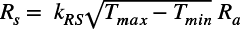(2)

where Tmax is maximum air temperature (ºC), Tmin is minimum air temperature (ºC), kRS is adjustment coefficient (0.16- 0.19).

#### 2.2.2 Support Vector Machine (SVM)

Vapnik (1995) developed the SVM method to solve pattern recognition and classification problems (Cortes and Vapnik, 1995). SVM is based on statistical learning theory and is mainly used to distinguish two data classes in the best possible way. For this purpose, decision boundaries or hyperplanes are determined. In a nonlinear dataset, SVMs cannot plot a linear hyperplane. Therefore, kernel numerals are used. The Kernel method widely increases machine learning on nonlinear data. The SVM estimator (y) process in the Kernel method is expressed as in Equation 3.(3)

where the Kernel core function is Kx,i, b is the bias term of the SVM network, and Wjk is called the weight vector. Kx and W represent Lagrange multipliers. Kx,i is a nonlinear function that maps input vectors to a high-dimensional feature space. Various function solutions determine the kernel core (Kx,i). In this study, the kernel function for the SVM method was determined as polynomial due to the lack of linearity between the input and output data. In the SVM method, the ‘Batch size’ value is 100, and the ‘Complexity’ value is 0.5.

#### 2.2.3 Artificial Neural Networks (ANN)

ANN is a mathematical modeling method whose development was inspired by biological neural systems like the human brain. In general, ANN is a system consisting of non-linear artificial cells that can be arranged as single-layer or multi-layer and work in parallel (Liu et al., 2010). The basic unit of ANN is the artificial neurons. Neurons are structures that produce a response through input nodes and process information within themselves. Artificial neurons have five components: inputs, weights, combination function, activation function, and outputs (Cıtakoglu and Ozeren, 2021). Artificial neurons get together to create ANN. The most common structure of ANN consists of the input layer, where the data is presented to the artificial neural network as input data; the hidden layer, where the data is processed; and the output layer, where the result is obtained. The basic structure of ANN is given in Figure 2.Fig. 2. Artificial Neural Networks Architecture

ANN has been the most important tool in solving complex nonlinear problems. ANN is widely used in hydrological studies such as precipitation-flow models, precipitation, runoff, temperature, snowmelt, evapotranspiration, solar radiation, and drought. (Khotanzad et al, 1996; Dawson and Wilby, 1998; Machado et al, 2011). This study used four hidden layers for the ANN model, 20 neurons in each hidden layer, the hyperbolic tangent function as the activation function, and the Levenberg-Marquardt algorithm as the training algorithm.

#### 2.2.4 Long Short-Term Memory Networks (LSTM)

LSTM, a type of recurrent neural network (RNN) approach, was first proposed by Hochreiter and Schmidhuber (1997a). Many people then developed the method. The LSTM model was specifically designed to overcome the exploding and disappearing gradient problems that typically arise when learning long-term dependencies, even if the minimum time delays are very long (Hochreiter and Schmidhuber, 1997b; Van Houdt et al., 2020). The LSTM unit consists of a cell, an input gate, an exit gate, and a forget gate. The structure of LSTM is given in Figure 3. These gates handle the writing, reading, and resetting of the cell. The cell remembers values at random time intervals, and three gates regulate the information flow associated with the cell (Singh et al., 2017). In the LSTM network structure, the input data is multiplied by the output of the input gate to identify new information that can be accumulated in the cell. To calculate the information that can be propagated to the network, the output data of the network is multiplied by the activation of the output gate. The cell states of the previous time are multiplied by the activation of the forget gate to determine whether the last state of the cell is forgotten. (Sainath et al., 2015).Fig. 3. LSTM Block (Sagheer and Kotb, 2019)

(Xt) is an input at time step t, the hidden state from the previous time step (St–1) is introduced to the LSTM block, and the hidden state (St) is then calculated as follows:

The first step; is to decide what information to discard from the cell state. This decision is made by the following forget gate (ft):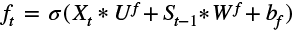(4)

The second step; is to decide what new information to store in the cell state. There are two stages in this step.

1. The input gate (it) layer decides which values to update.
2. The tanh function determines the candidate information that will create new information.(5)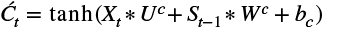(6)

The third step, from the old cell state Ct−1 is to create the new cell state Ct as follows.(7)

The final step decides what will be produced as output. The output gate (Ot) determines which components of the cell state will be generated as output in this stage. Then the cell state goes through the tanh layer (to push between -1 and 1 the values) and multiplies it with the output gate as follows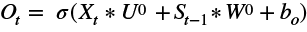(8)(9)

From the previous six equations, the LSTM presents the following three groups of parameters:

1. Input weights: Uf, Ui, Uc, U0.
2. Recurrent weights: Wf, Wi, Wc, W0
3. Bias: bf, bc, bi, bo.

The LSTM model used in the study has one input layer, one hidden layer containing 64 LSTM neurons, and one output layer that makes a single value estimation. Sigmoid was used as the activation function in LSTM neurons. The learning rate of the model was determined as 0.005. The dropout value is set to 0.1 to avoid over-fitting. The stochastic Gradient Descent (SGD) algorithm was used as the optimization method. The LSTM model is trained for 100 epochs, and one batch size is used in this training (Li and Cao, 2018; Granata and Di Nunno, 2021).

#### 2.3. Predictive Model Development

In this study, solar radiation values were estimated by giving input attributes determined for the Hargreaves-Samani equation and Angstrom equation as input to machine learning and deep learning model. Since the input parameters of both equations were different, two different models were created. The input attributes for the Hargreaves-Samani equation were extraterrestrial radiation, daily minimum air temperature, and daily maximum air temperature. The input attributes for the Angstrom equation were the extraterrestrial radiation, the actual sunshine duration, and the maximum duration of sunshine hours. Extraterrestrial radiation was determined from solar constant, solar declination, and time of year for different time periods and latitudes by the method specified in Allen et al. (1998). In estimating the solar radiation value, which is the dependent variable, performance comparisons of ANN, SVM, and LSTM methods are made for both equations. In addition, the values calculated by the Hargreaves-Samani equation and Angstrom equation, which are used to complete the missing data in the solar radiation values measured in the literature, were compared with the estimated values of machine learning and deep learning models. In this study, the kernel function for the SVM method was determined as polynomial due to the lack of linearity between the input and output data. In the SVM method, the ‘Batch size’ value is 100, and the ‘Complexity’ value is 0.5. The most critical disadvantage of ANN and LSTM methods is that there is no standard way to determine model parameters (Sherstinsky, 2020). The optimum parameters were determined in the study by the trial-and-error method until the most suitable values for these two methods were found. For the ANN model, four hidden layers, 20 neurons in each hidden layer, hyperbolic tangent function as activation function, and Levenberg-Marquardt algorithm as training algorithm was used. The LSTM model used in the study has one input layer, one hidden layer containing 64 LSTM neurons, and one output layer that makes a single value estimation. Sigmoid was used as the activation function in LSTM neurons. The learning rate of the model was determined as 0.005. The dropout value is set to 0.1 to avoid over-fitting. The Stochastic Gradient Descent (SGD) algorithm was used as the optimization method. The LSTM model is trained for 100 epochs, and one batch size is used in this training (Li and Cao, 2018; Granata and Di Nunno, 2021).

It is thought that a dataset containing a total of 2112 daily solar radiation values is sufficient for objectively evaluating the machine learning and deep learning models used in the study (Gers et al., 2000). The study applied a 3-fold cross-validation method to the dataset prepared for the solar radiation value estimation, allowing objective evaluation of ANN, SVM, and LSTM models (Hastie et al., 2009). This method divides the dataset into two sets, 80% of the values for training (1691) and 20% for testing (421). The study’s dataset was changed in the same distribution ratios, and three different test results were found. The experiments were carried out by taking the average of these results (Kohavi, 1995).

#### 2.4 Model performance measures

In this study, mean absolute error (MAE), root means square error (RMSE), mean relative error (MRE), and determination coefficient (R2) were used as performance evaluation criteria of the models. The fact that the MAE value, which questions the absolute error between the real and the estimated values, is close to zero reveals the result of the model’s good estimation ability (Duan et al., 2016).(10)

In Equation 10, x is the actual value, x’ is the estimated value, and n is the number of data. While calculating the RMSE value, the sum of the squares of the errors of the data set is divided by the number of data, and the square root of this value is taken (Duan et al., 2016).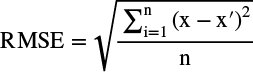(11)

Since the errors are squared in the RMSE value, large errors in the data set significantly affect the mean. In this way, it is ensured that large errors have a greater effect on the overall evaluation. The fact that the RMSE value is generally below 10% of the mean dependent variable value reveals the result of a successful estimation (Lee, 2014). The MRE value calculates the relative absolute error between the actual values and the estimated values.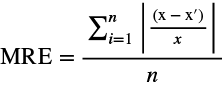(12)

The fact that the MRE value given by Equation 12 approaches zero indicates that the estimative ability of the model is good. The R2 value, which represents the rate of variance of the dependent variable explained by the independent variables, is defined in the range of 0 to 1. A close to zero value indicates no relationship between the independent and dependent variables. In contrast, a value close to one shows that the independent variables can explain the dependent variables. The value is desired to be close to one (Fisher, 1922).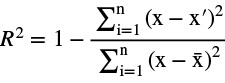(13)

3. Results and Discussion

The average MAE, RMSE, MRE, and R2 values obtained from the experiments performed for the Hargreaves-Samani and the Angstrom equation are given in Tables II and III.

Table II. Average estimation results of solar radiation values from the Hargreaves-Samani equation

 Solar Radiation Value Estimation Method Evaluation Criteria R2 MAE RMSE MRE Hargreaves-Samani 0.9236 2.65 3.398 0.312 SVM 0.964 1.257 1.628 0.148 ANN 0.9685 1.183 1.512 0.136 LSTM 0.9749 0.987 1.298 0.109

Table III. Average estimation results of solar radiation values from the Angstrom equation

 Solar Radiation Value Estimation Method Evaluation Criteria R2 MAE RMSE MRE Angstrom 0.9645 1.24 1.624 0.135 SVM 0.9699 1.02 1.465 0.122 ANN 0.9768 0.87 1.25 0.104 LSTM 0.9806 0.747 1.073 0.089

As can be seen in Tables II and III, two different models created with input from the Hargreaves-Samani and Angstrom equations for the LSTM model are the most successful among machine learning and deep learning methods used in experimental studies. The results obtained with machine learning and deep learning models show more successful outcomes than the solar radiation values calculated by both the Hargreaves-Samani and the Angstrom equations alone. The results obtained with input from the Angstrom equation are more successful than the results with input from the Hargreaves-Samani equation. MAE, RMSE, and MRE values approaching zero and R2 values approaching one indicate the success of our proposed model. The graphical representation of the absolute errors in the test dataset for solar radiation value estimation using the LSTM deep learning model, for the most successful model with the Hargreaves-Samani equation, is shown in Figure 4.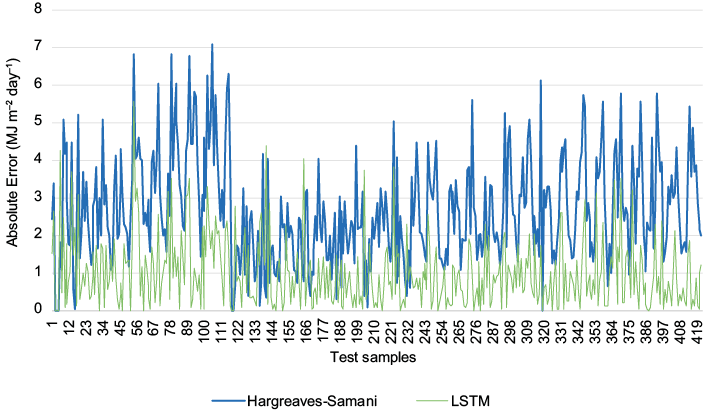Fig. 4. Graphical representation of absolute errors of the Hargreaves-Samani equation and LSTM model

As seen in Figure 4, the Hargreaves-Samani equation and LSTM deep learning model were compared according to the monthly solar radiation value estimation results with a dataset consisting of 2112 records and eight attributes. The LSTM deep learning model was observed to be more successful than the Hargreaves-Samani equation and minimizes the estimation error. It was observed that the MAE value, which was 2.65 with the Hargreaves-Samani equation, decreased to 0.987 with the LSTM model.

The graphical representation of the absolute errors in the test dataset for solar radiation value estimation using the LSTM deep learning model, for the most successful model with the Angstrom equation, is shown in Figure 5.Fig. 5. Graphical representation of absolute errors of the Angstrom equation and LSTM model

As seen in Figure 5, the LSTM deep learning model was also more successful than the Angstrom equation and minimized the estimation error. As a result of the experimental studies, although linearity is observed between the solar radiation values and the input data set, it was observed that the error value was reduced by using SVM and ANN. In this situation, the LSTM model, one of the deep learning methods, was applied, and it was observed that the MAE value, which was 1.24, decreased to 0.747 with the Angstrom equation. In addition, although the solar radiation estimation calculated using the Angstrom equation seemed more successful than the Hargreaves-Samani equation, the LSTM model showed more successful results than both equations. The test samples shown in Figures 4 and 5 were randomly taken from six different stations. The random sampling of the test samples showed that the data collection periods were also random. This is especially important in terms of objective evaluation of the results.

Figure 6 and Figure 7 showed box-whisker error plots corresponding to the absolute values of the difference between solar radiation values calculated from the Hargreaves-Samani and Angstrom equations and estimated values from the models. In this way, the distribution characteristics of the errors were revealed, and the models were compared objectively (Di Nunno et al., 2021). The line in the middle of the box in the box-whisker plot shows the median value (Q2), the lower boundary line of the box indicates the first quartile (Q1), and the upper boundary line of the box indicates the third quartile (Q3). As the Q3Q1 (IQR-interquartile range) value increases, the spread of the data increases, and as it gets smaller, it decreases. As the Q2Q1 value gets larger, the median value moves away from the first quarter, and the data set shows a left-skewed distribution. As the Q3Q2 value gets larger, the median value moves away from the third quartile, and the data set shows a right-skewed distribution. The data set exhibits a symmetrical distribution when the median, mean, and mode values are in the middle of the quadrants. The cross on the box gives the mean of the data set. Therefore, it shows the MAE value. The vertical lines coming out of the boxes denote whiskers. The lower whisker or lower line represents the smallest (minimum), and the upper whisker or upper line represents the largest (maximum) data value. The dot or dots outside the whiskers represent outliers. The position of the quadrants changes as the number of outliers and their spread beyond the whiskers increases. Therefore, when comparing multiple box-whisker plots, it is necessary to consider the number of outliers, their spread, the box width, and the whiskers’ lengths.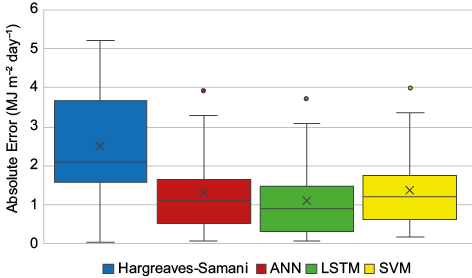Fig. 6. Boxplot of Hargreaves-Samani, ANN, LSTM, SVM absolute errors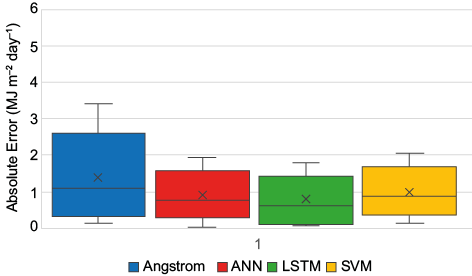Fig. 7. Boxplot of Angstrom, ANN, LSTM, SVM absolute errors

Figure 6 illustrates that while the Hargreaves-Samani model does not contain outliers, the ANN, LSTM, and SVM models do. Although the Hargreaves-Samani model appears to be more favorable in this regard, it is clear that the LSTM model stands out when compared to the others when looking at whisker lengths, box sizes, MAE values, and how far these values are from the quarters. On the other hand, the Hargreaves-Samani model exhibits a right-skewed structure, and the SVM model exhibits a left-skewed structure. ANN and LSTM models show a more symmetrical structure. Considering the whisker lengths, LSTM can be preferred over the ANN model.

As seen in Figure 7, although all models show a right-skewed distribution, the size of the boxes and whisker lengths in the Angstrom model showed a wider spread than the ANN, LSTM, and SVM models. Although the box widths of the ANN, LSTM, and SVM models seem the same, the smallest data value in the LSTM model almost coincided with the Q1 quartile. This situation caused the MAE value to be even smaller. Therefore, in terms of the distribution of absolute error values, the most stable model seems to be LSTM.

4. Conclusion

This study has developed LSTM deep learning, ANN, and SVM machine learning models to estimate Turkey’s monthly solar radiation value. Training and testing processes were carried out with the meteorological data of a total of six stations in Turkey that receive the most and the least solar radiation. When the developed and empirical models are evaluated together, it is concluded that all learning models make more successful estimations than empirical models. While the LSTM model has lower MAE, RMSE, and MRE values than other learning models and empirical models, it has a higher R2 value. Therefore, the best-performing method is the LSTM deep learning model. As a result, experts who perform solar radiation calculations can successfully estimate solar radiation with the LSTM model in cases where measurement is impossible.

This study, which deals with estimating the solar radiation value, transfers the computational engineering task from the researchers to the deep learning model. The deep learning approach performs much better when comparing the deep learning method, the empirical models, and the traditional machine learning models. The success of the deep learning approach is based on two main reasons. The first is that deep learning models mimic how the human brain works. High-level attributes that represent semantic relationships are extracted by taking the lower-level attributes with the successive layers of the model. Second, thanks to the memory module in the LSTM model, it automatically identifies active features at each step of the training. Finally, for the estimation process used in this study, ANN, SVM, and LSTM machine learning/deep learning methods seem to be methods that can be used in relational screening studies. These methods are known to be powerful estimation methods. For this reason, it is recommended that these methods be used more widely in similar studies to be conducted in the future.

References

Allen RG, Pereira LS, Raes D, Smith M. 1998. Crop evapotranspiration: Guidelines for computing crop water requirements. FAO Irrigation and Drainage Paper 56. United Nations Food and Agriculture Organization. Rome, Italy, 300 pp

Amrouche B, Le Pivert X. 2014. Artificial neural network based daily local forecasting for global solar radiation. Applied Energy 130: 333-341. https://doi.org/10.1016/j.apenergy.2014.05.055

Angstrom A. 1924. Solar and terrestrial radiation. Report to the international commission for solar research on actinometric investigations of solar and atmospheric radiation. Quarterly Journal of Royal Meteorological Society 50: 121-126. https://doi.org/10.1002/qj.49705021008

Atalay İ. 2011. Türkiye Coğrafyası ve Jeopolitiği (8.Baskı). İzmir: Meta Basım Matbaacılık Hizmetleri.

Besharat F, Dehghan AA, Faghih AR. 2013. Empirical models for estimating global solar radiation: a review and case study. Renewable and Sustainable Energy Reviews 21: 798-821. https://doi.org/10.1016/j.rser.2012.12.043

Çelik S. 2020. Turkey’s Geopolitical Position Today. The Journal of International Social Research 13: 202-210.

Chandola D, Gupta H, Tikkiwal VA, Bohra MK. 2020. Multi-step ahead forecasting of global solar radiation for arid zones using deep learning. Procedia Computer Science 167: 626–635. https://doi.org/10.1016/j.procs.2020.03.329

Chen JL, Liu HB, Wu W, Xie DT. 2011. Estimation of monthly solar radiation from measured temperatures using support vector machines – A case study. Renewable Energy 36: 413-420. https://doi.org/10.1016/j.renene.2010.06.024

Cıtakoglu H, Ozeren Y. 2021. Sakarya Basin Water Quality Parameters Modeling with Artificial Neural Networks. European Journal of Science and Technology Special Issue 24, 10-17. https://doi.org/10.31590/ejosat.898046

Cortes C, Vapnik V. 1995. Support-vector networks. Machine Learning 20: 273-297. https://doi.org/10.1007/BF00994018

Dawson CW, Wilby R. 1998. An artificial neural network approach to rainfall-runoff modelling. Hydrological Sciences Journal 43: 47-66. https://doi.org/10.1080/02626669809492102

Di Nunno F, Granata F, Gargano R, de Marinis G. 2021. Prediction of spring flows using nonlinear autoregressive exogenous (NARX) neural network models. Environmental Monitoring and Assessment 193: 350. https://doi.org/10.1007/s10661-021-09135-6

Duan Y, Yisheng LV, Wang FY. 2016. Travel time prediction with LSTM neural network. In: 2016 IEEE 19th International Conference on Intelligent Transportation Systems (ITSC): 1053-1058. https://doi.org/10.1109/ITSC.2016.7795686

Fisher RA. 1922. The Goodness of Fit of Regression Formulae, and the Distribution of Regression Coefficients. Journal of the Royal Statistical Society 85: 597-612. https://doi.org/10.2307/2341124

Gers FA, Schmidhuber J, Cummins F. 2000. Learning to Forget: Continual prediction with LSTM. Neural computation 12: 2451-2471. https://doi.org/10.1162/089976600300015015

Granata F, Di Nunno F. 2021. Forecasting evapotranspiration in different climates using ensembles of recurrent neural networks. Agricultural Water Management 255: 107040, https://doi.org/10.1016/j.agwat.2021.107040

Guermoui M, Rabehi A. 2018. Soft computing for solar radiation potential assessment in Algeria. International Journal of Ambient Energy 41: 1524-1533. https://doi.org/10.1080/01430750.2018.1517686

Hargreaves GH, Samani ZA. 1982. Estimating Potential Evapotranspiration. Journal of Irrigation and Drainage Division 108: 223− 230. https://doi.org/10.1061/JRCEA4.0001390

Hassan GE, Youssef ME, Mohamed ZE, Ali MA, Hanafy AA. 2016. New Temperature-based Models for Predicting Global Solar Radiation. Applied Energy 179: 437-450. https://doi.org/10.1016/j.apenergy.2016.07.006

Hastie T, Tibshirani R, Friedman J. 2009. Unsupervised learning. In: The Elements of Statistical Learning: Data Mining, Inference, and Prediction. New York: Springer New York. https://doi.org/10.1007/978-0-387-84858-7_14

Hochreiter S, Schmidhuber J. 1997a. Long Short-Term memory. Neural Computation 9: 1735-1780. https://doi.org/10.1162/neco.1997.9.8.1735

Hochreiter S, Schmidhuber J. 1997b. LSTM can solve hard long time lag problems. In: Mozer MC, Jordan M, Petsche T, eds. Advances in Neural Information Processing Systems 9, 473-479.

Van Houdt G, Mosquera C, Nápoles G. 2020. A review on the long short-term memory model. Artificial Intelligence Review 53: 5929–5955. https://doi.org/10.1007/s10462-020-09838-1

Jiang Y. 2009. Computation of monthly mean daily global solar radiation in China using artificial neural networks and comparison with other empirical models. Energy 34: 1276-1283. https://doi.org/10.1016/j.energy.2009.05.009

Khotanzad A, Davis MH, Abaye A, Maratukulam DJ. 1996. An artificial neural network hourly temperature forecaster with applications in load forecastin. IEEE Transactions on Power Systems 11: 870-876. https://doi.org/10.1109/59.496168

Kohavi R. 1995. A study of cross-validation and bootstrap for accuracy estimation and model selection. In: Proceedings of the 14th International Joint Conference on Artificial Intelligence, Vol. 2. Montreal 1137-1145.

Lauret P, Voyant C, Soubdhan T, David M, Poggi P. 2015. A benchmarking of machine learning techniques for solar radiation forecasting in an insular context. Solar Energy 112: 446–457. https://doi.org/10.1016/j.solener.2014.12.014

Lee PH. 2014. Is a Cutoff of 10% Appropriate for the Change-in-Estimate Criterion of Confounder Identification? Journal of Epidemiology 24: 161-167. https://doi.org/10.2188/jea.JE20130062

Li YF, Cao H. 2018. Prediction for Tourism Flow based on LSTM Neural Network. Procedia Computer Science 129: 277-283. https://doi.org/10.1016/j.procs.2018.03.076

Liu ZL, Peng CH, Xiang WH, Tian DL, Deng XW, Zhao MF. 2010. Application of artificial neural networks in global climate change and ecological research: An overview. Chinese Science Bulletin 55: 3853–3863. https://doi.org/10.1007/s11434-010-4183-3

Machado F, Mine M, Kaviski E, Fill H. 2011. Monthly rainfall–runoff modelling using artificial neural networks. Hydrological Sciences Journal 56: 349-361. https://doi.org/10.1080/02626667.2011.559949

Mızrak G. 1983. Türkiye Iklim Bölgeleri ve Haritası. Teknik Yayınlar No: 2. Genel Yayın No:52. Ankara, Turkey: Orta Anadolu Bölge Zirai Araştırma Enstitüsü.

Mohammadi B, Moazenzadeh R. 2021. Performance Analysis of Daily Global Solar Radiation Models in Peru by Regression Analysis. Atmosphere 12: 389. https://doi.org/10.3390/atmos12030389

Pereira LS, Allen RG, Smith M, Raes D. 2015. Crop evapotranspiration estimation with FAO56: Past and future. Agricultural Water Management 147: 4–20. https://doi.org/10.1016/j.agwat.2014.07.031

Premalatha N, Valan Arasu A. 2016. Prediction of solar radiation for solar systems by using ANN models with different back propagation algorithms. Journal of Applied Research and Technology 14: 206-214. https://doi.org/10.1016/j.jart.2016.05.001

Rahimikhoob A. 2010. Estimating global solar radiation using artificial neural network and air temperature data in a semi-arid environment. Renewable Energy 35: 2131–2135. https://doi.org/10.1016/j.renene.2010.01.029

Sagheer A, Kotb M. 2019. Time series forecasting of petroleum production using deep LSTM recurrent networks. Neurocomputing 323: 203-213. https://doi.org/10.1016/j.neucom.2018.09.082

Sainath TN, Vinyals O, Senior A, Sak H. 2015. Convolutional, Long Short-Term Memory, fully connected Deep Neural Networks. In: IEEE International Conference on Acoustics, Speech and Signal Processing. Brisbane, Australia, 4580-4584. https://doi.org/10.1109/ICASSP.2015.7178838

Shamshirband S, Mohammadi K, Chen HL, Narayana Samy G, Petković D, Ma C. 2015. Daily global solar radiation prediction from air temperatures using kernel extreme learning machine: A case study for Iran. Journal of Atmospheric and Solar-Terrestrial Physics 134: 109-117. https://doi.org/10.1016/j.jastp.2015.09.014

Sherstinsky A. 2020. Fundamentals of Recurrent Neural Network (RNN) and Long Short-Term Memory (LSTM) network. Physica D: Nonlinear Phenomena 404: 132306. https://doi.org/10.1016/j.physd.2019.132306

Singh D, Merdivan E, Psychoula I, Kropf J, Hanke S, Geist M, Holzinger A. 2017. Human activity recognition using recurrent neural networks. In: Holzinger A, Kieseberg P, Tjoa A, Weippl, E, eds. International Cross-Domain Conference for Machine Learning and Knowledge Extraction. CD-Make 2017, 267-274. https://doi.org/10.1007/978-3-319-66808-6_18

Sözen A, Arcaklıoğlu E, Özalp M. 2004. Estimation of solar potential in Turkey by artificial neural networks using meteorological and geographical data. Energy Conversion and Management 45: 3033-3052. https://doi.org/10.1016/j.enconman.2003.12.020

Sözen A, Arcaklıoğlu E, Özalp M, Çağlar N. 2005. Forecasting based on neural network approachof solar potential in Turkey. Renewable Energy 30: 1075-1090. https://doi.org/10.1016/j.renene.2004.09.020

Vapnik VN. 1995. The Nature of Statistical Learning Theory. New York: Springer New York. https://doi.org/10.1007/978-1-4757-3264-1

Yacef R, Benghanem M, Mellit A. 2012. Prediction of daily global solar irradiation data using Bayesian neural network: A comparative study. Renewable Energy 48: 146-154, https://doi.org/10.1016/j.renene.2012.04.036

Yadav AK, Chandel SS. 2014. Solar radiation prediction using Artificial Neural Network Techniques: A review. Renewable and Sustainable Energy Reviews 33: 772–781. http://doi.org/10.1016/j.rser.2013.08.055

Yang K, Koike T, Ye B. 2006. Improving estimation of hourly, daily, and monthly solar radiation by importing global data sets. Agricultural and Forest Meteorology 137: 43-55. https://doi.org/10.1016/j.agrformet.2006.02.001

Yıldırım HB, Teke A, Antonanzas-Torres F. 2018. Evaluation of classical parametric models for estimating solar radiation in the Eastern Mediterranean region of Turkey. Renewable and Sustainable Energy Reviews 82: 2053–2065. https://doi.org/10.1016/j.rser.2017.08.033

Yurekli K. 2021. Scrutinizing variability in full and partial rainfall time series by different approaches. Natural Hazards 105: 2523–2542. https://doi.org/10.1007/s11069-020-04410-0

Zanetti, SS, Dohler, RE, Cecílio, RA, Pezzopane, JEM, Xavier, AC. 2019. Proposal for the use of daily thermal amplitude for the calibration of the Hargreaves-Samani equation. Journal of Hydrology 571: 193–201. https://doi.org/10.1016/j.jhydrol.2019.01.049